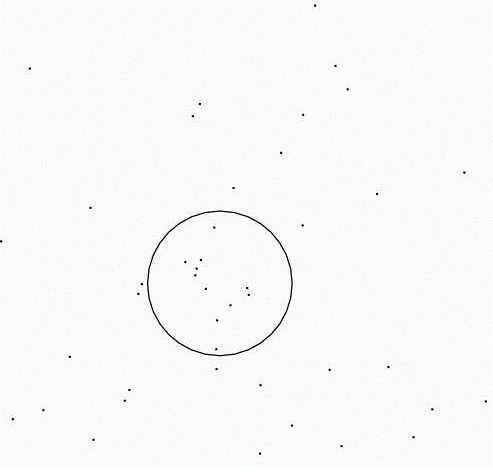Online JudgeProblem SetAuthorsOnline ContestsUser
Web Board
F.A.Qs
Statistical Charts
Problems
Submit Problem
Online Status
Prob.ID:
Register
Authors ranklist
Current Contest
Past Contests
Scheduled Contests
Award Contest
Register
Language:
Circle and Points
 Time Limit: 5000MS Memory Limit: 30000K Total Submissions: 9578 Accepted: 3414 Case Time Limit: 2000MS

Description

You are given N points in the xy-plane. You have a circle of radius one and move it on the xy-plane, so as to enclose as many of the points as possible. Find how many points can be simultaneously enclosed at the maximum. A point is considered enclosed by a circle when it is inside or on the circle.Fig 1. Circle and Points

Input

The input consists of a series of data sets, followed by a single line only containing a single character '0', which indicates the end of the input. Each data set begins with a line containing an integer N, which indicates the number of points in the data set. It is followed by N lines describing the coordinates of the points. Each of the N lines has two decimal fractions X and Y, describing the x- and y-coordinates of a point, respectively. They are given with five digits after the decimal point.

You may assume 1 <= N <= 300, 0.0 <= X <= 10.0, and 0.0 <= Y <= 10.0. No two points are closer than 0.0001. No two points in a data set are approximately at a distance of 2.0. More precisely, for any two points in a data set, the distance d between the two never satisfies 1.9999 <= d <= 2.0001. Finally, no three points in a data set are simultaneously very close to a single circle of radius one. More precisely, let P1, P2, and P3 be any three points in a data set, and d1, d2, and d3 the distances from an arbitrarily selected point in the xy-plane to each of them respectively. Then it never simultaneously holds that 0.9999 <= di <= 1.0001 (i = 1, 2, 3).

Output

For each data set, print a single line containing the maximum number of points in the data set that can be simultaneously enclosed by a circle of radius one. No other characters including leading and trailing spaces should be printed.

Sample Input

```3
6.47634 7.69628
5.16828 4.79915
6.69533 6.20378
6
7.15296 4.08328
6.50827 2.69466
5.91219 3.86661
5.29853 4.16097
6.10838 3.46039
6.34060 2.41599
8
7.90650 4.01746
4.10998 4.18354
4.67289 4.01887
6.33885 4.28388
4.98106 3.82728
5.12379 5.16473
7.84664 4.67693
4.02776 3.87990
20
6.65128 5.47490
6.42743 6.26189
6.35864 4.61611
6.59020 4.54228
4.43967 5.70059
4.38226 5.70536
5.50755 6.18163
7.41971 6.13668
6.71936 3.04496
5.61832 4.23857
5.99424 4.29328
5.60961 4.32998
6.82242 5.79683
5.44693 3.82724
6.70906 3.65736
7.89087 5.68000
6.23300 4.59530
5.92401 4.92329
6.24168 3.81389
6.22671 3.62210
0
```

Sample Output

```2
5
5
11
```

Source

[Submit]   [Go Back]   [Status]   [Discuss]Home PageGo BackTo top